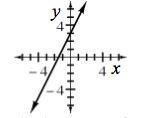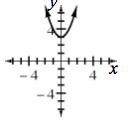Home > A2C > Chapter 1 > Lesson 1.2.2 > Problem1-97

1-97.

Graph the following equations.

Put in $y=$ form first. Make a table if you cannot remember your graphing shortcuts.

1. $y−2x=3$1. $y−3=x^2$1. State the $x$- and $y$-intercepts for each equation.

$\text{a}: x\text{-intercept}=\left(-\frac{3}{2},0\right);\ y\text{-intercept} = (0, 3)$

$\text{b}:\text{ no }x\text{-intercept; }y\text{-intercept }=(0, 3)$

1. Where do the two graphs cross? Show how you can find these two points without looking at the graphs.

Solve for the point of intersection algebraically.

$2x+3=x^2+3$